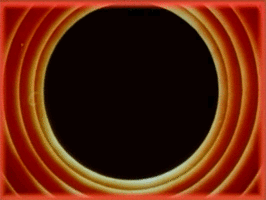# Poisson Disk Sampling in Processing

If you’re looking for a copy-pastable version of this algorithm in Processing, scroll to the end of the post!

What is Poisson Disk Sampling and why should you care? Poisson disk sampling is a technique for randomly picking tightly-packed points such that they maintain a minimum user-specified distance. Since points are randomly picked, the result still has an organic feel to it. Below is an example showing the set of chosen points.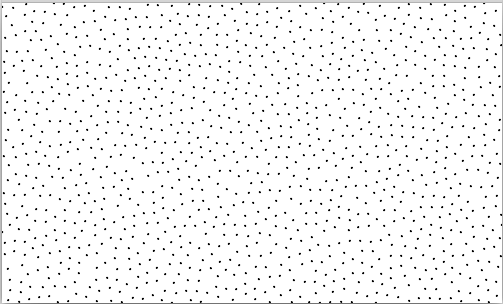## Bridson’s Algorithm

In this post, we’re going to implement a Poisson Disk Sampling algorithm in Processing. Specifically, we’re going to implement a fast algorithm for doing this as described in this short, very readable, one-page paper by Robert Bridson. In fact, in the paper, the algorithm itself is described in its entirety as shown below. In this post, I’ll break it down step-by-step and show you how to transform this high-level description into code. Don’t be scared!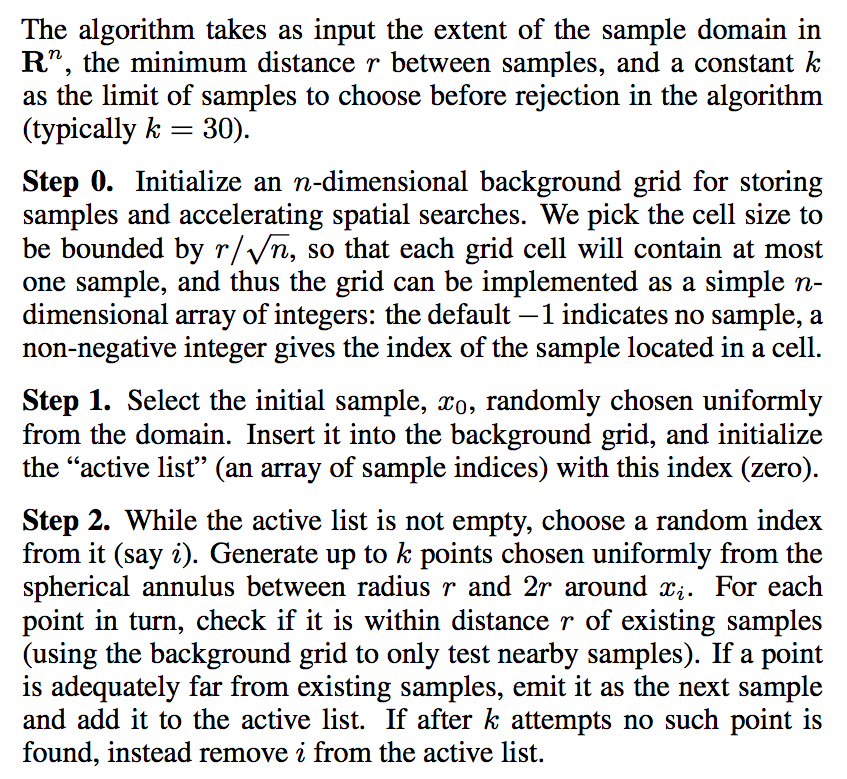Here is an animation of Bridson’s Poisson Disk Sampling algorithm at work.## The Input to the Algorithm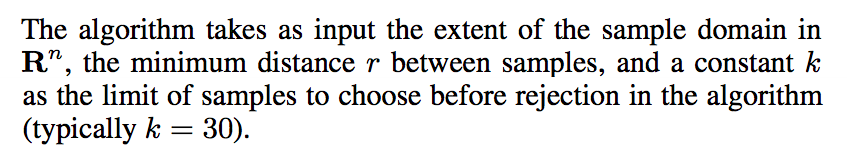There are three pieces of information we give as input to the algorithm, as described in the paper: (i) the dimension of the samples we want (in our case, for a 2D screen, N=2), (ii) the minimum distance between samples and (iii) a limit k. The algorithm returns a list of points. Based on this, we can already define a skeleton function as shown below:

ArrayList<PVector> poissonDiskSampling(float radius, int k) {
int N = 2;
ArrayList<PVector> points = new ArrayList<PVector>();

/* Code goes here */

return points;
}


The function takes as parameters the minimum distance (radius) and the limit k, and returns a list of points (we represent this using an ArrayList<PVector> for convenience). Since N is fixed, we don’t need to pass it as a parameter, and just define a constant.

Not so bad so far right?

## Step 0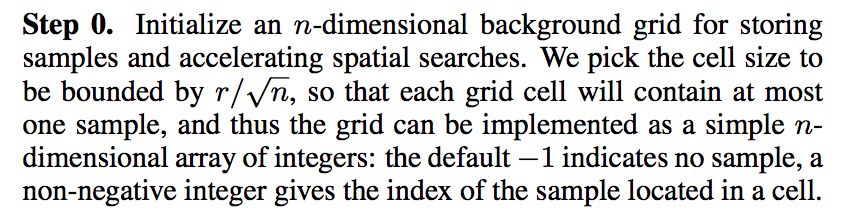We need to initialize an N-dimensional grid of squares. Recall that in our case, N=2; so we just need to create a 2D grid. The paper tells us that the size of each cell in the grid should be r/sqrt(N). Why this number? Why do we need this weird grid to begin with?

### Understanding the Cell Size

Again, the answer is in the writing: we want to use the grid to reduce our search area down so that the algorithm progresses quickly. And to make it even faster, we’re going to pick a cell size in such a way that we’re guaranteed to have at most one point in any given cell.

So why r/sqrt(2)?

What we want is that the longest possible distance between two circles in adjacent cells never exceeds our user-specified radius. Let’s take the worst case: we end up picking one of the corners as our point for that cell, and the opposite corner in the next iteration (this is the worst case because the diagonal is the longest possible distance within a circle).

So if we have the side of our square grid cell of some unknown length x, we want the diagonal to be at most r. However, Pythagoras’ theorem tells us that the for the diagonal in a square with side x, we have r^2 = x^2 + x^2.

Solving for x, we get the value r/sqrt(2).

What this means is that if we have adjacent square cells with sides of that length, then we are guaranteed to never have two points in the same cell!

### Implementing the Grid

Now we can implement the grid very easily using a 2D array of PVector’s.

ArrayList<PVector> poissonDiskSampling(float radius, int k) {
int N = 2;
ArrayList<PVector> points = new ArrayList<PVector>();

PVector[][] grid;

/* Figure out no. of cells in the grid for our canvas */
int ncells_width = ceil(width/cellsize) + 1;
int ncells_height = ceil(height/cellsize) + 1;

/* Allocate the grid an initialize all elements to null */
grid = new PVector[ncells_width][ncells_height];
for (int i = 0; i < ncells_width; i++)
for (int j = 0; j < ncells_height; j++)
grid[i][j] = null;

/* Step 1 and 2 go here */

return points;
}


Notice that in our case, we slightly modify the description of the algorithm. Instead of storing indices into a point array, we just store the PVector object itself. Similarly, instead of initializing everything to -1, we initialize each cell to null.

Let’s also quickly write a function to insert a point into the grid.

void insertPoint(PVector[][] grid, PVector point) {
int xindex = floor(point.x / cellsize);
int yindex = floor(point.y / cellsize);
grid[xindex][yindex] = point;
}


We calculate which cell our point should fall in by dividing its coordinates by the cell size, and then set that value in the grid.

And that’s it! We have ourselves a grid with a handy function to add a point into it!

## Step 1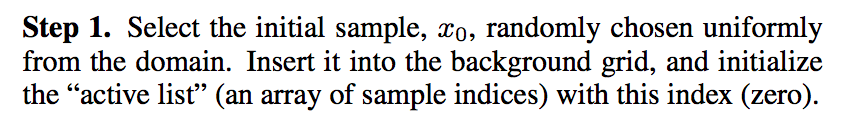We now need to pick an initial point on the canvas. We then insert it into the background grid. However, we also need to initialize an “active list” of points with this first point.

Let’s quickly modify our function to create an initial random point (p0 in the code below), as well as the “active list” (active in the code below):

ArrayList<PVector> poissonDiskSampling(float radius, int k) {
int N = 2;
/* The final set of points to return */
ArrayList<PVector> points = new ArrayList<PVector>();
/* The currently "active" set of points */
ArrayList<PVector> active = new ArrayList<PVector>();
/* Initial point p0 */
PVector p0 = new PVector(random(width), random(height));
...
/* Earlier grid initialization code here */
...
insertPoint(grid, p0);

/* Steps 2 and 3 go here */

return points;
}


Above, we first allocate an active list and an initial random point. And following our earlier grid initialization code, we insert this point into the grid as well as our two lists.

## Step 2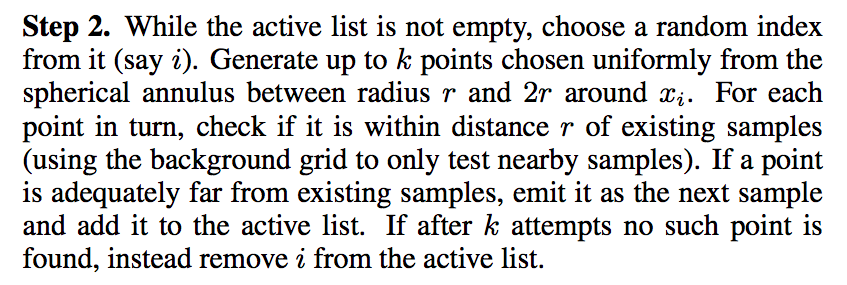To understand step 2 better, I would suggest looking at this animation here first.

The core of the algorithm is a loop that continues until the active list is emptied.

ArrayList<PVector> poissonDiskSampling(float radius, int k) {
...
while (active.size() > 0) {
/* Pick a point 'p' from our active list */

/* Try up to 'k' times to find a point that satisfies:
* -- Is at a distance between r and 2r from p
* -- Is at a distance > r from nearby points
*/

/* If we succeed in finding a point, add to grid and lists */

/* Otherwise, remove point 'p' from the active list */
}
...
}


Let’s take each of these steps one at a time. Picking a point is of course easy:

int random_index = int(random(active.size()));
PVector p = active.get(random_index);


We now set a boolean flag to know if the k tries succeeded. We initilize it to false and only set it to true in the loop if we find a valid point. If after the loop, found is still false, remove our picked point from the active list.

ArrayList<PVector> poissonDiskSampling(float radius, int k) {
...
while (active.size() > 0) {
int random_index = int(random(active.size()));
PVector p = active.get(random_index);

boolean found = false;
for (int tries = 0; tries < k; tries++) {
/* If we find a valid point: set found = true */
}

/* If no point was found after k tries, remove p */
if (!found)
active.remove(idx);
}
...
}


Now let’s get to the meat of the inner loop where we try to create a new point.

### The Inner Loop

ArrayList<PVector> poissonDiskSampling(float radius, int k) {
...
while (active.size() > 0) {
...
for (int tries = 0; tries < k; tries++) {
/* 1. Create a new point */
/* 2. Check if it's a valid point */
/* 3. Add point and set 'found' if valid */
}
...
}
...
}


Let’s deal with steps 1 and 3 first since they’re fairly easy. The paper says that we should pick a random point at a distance between r and 2*r. We can do this as shown below.

/* Pick a random angle */
float theta = random(360);
/* Pick a random radius between r and 2r */
/* Find X & Y coordinates relative to point p */
PVector pnew = new PVector(pnewx, pnewy);


For step 3, we want to (i) add the valid point to the grid, active list and list of final points, and (ii) set found to true.

points.add(pnew);
insertPoint(grid, pnew);
found = true;
break;


Now let’s get to step 2. We want to basically check the nearby cells and make sure we’re not close to any pre-existing point. We can write a function to do this easily.

boolean isValidPoint(PVector[][] grid,
int gwidth, int gheight,
/* Make sure the point is on the screen */
if (p.x < 0 || p.x >= width || p.y < 0 || p.y >= height)
return false;

/* Check neighboring eight cells */
int xindex = floor(p.x / cellsize);
int yindex = floor(p.y / cellsize);
int i0 = max(xindex - 1, 0);
int i1 = min(xindex + 1, gwidth - 1);
int j0 = max(yindex - 1, 0);
int j1 = min(yindex + 1, gheight - 1);

for (int i = i0; i <= i1; i++)
for (int j = j0; j <= j1; j++)
if (grid[i][j] != null)
if (dist(grid[i][j].x, grid[i][j].y, p.x, p.y) < radius)
return false;

/* If we get here, return true */
return true;
}


And that’s it!

## Final Code

After all that code, here is the final result. A copy-pastable version for inclusion in your sketches!

boolean isValidPoint(PVector[][] grid, float cellsize,
int gwidth, int gheight,
/* Make sure the point is on the screen */
if (p.x < 0 || p.x >= width || p.y < 0 || p.y >= height)
return false;

/* Check neighboring eight cells */
int xindex = floor(p.x / cellsize);
int yindex = floor(p.y / cellsize);
int i0 = max(xindex - 1, 0);
int i1 = min(xindex + 1, gwidth - 1);
int j0 = max(yindex - 1, 0);
int j1 = min(yindex + 1, gheight - 1);

for (int i = i0; i <= i1; i++)
for (int j = j0; j <= j1; j++)
if (grid[i][j] != null)
if (dist(grid[i][j].x, grid[i][j].y, p.x, p.y) < radius)
return false;

/* If we get here, return true */
return true;
}

void insertPoint(PVector[][] grid, float cellsize, PVector point) {
int xindex = floor(point.x / cellsize);
int yindex = floor(point.y / cellsize);
grid[xindex][yindex] = point;
}

ArrayList<PVector> poissonDiskSampling(float radius, int k) {
int N = 2;
/* The final set of points to return */
ArrayList<PVector> points = new ArrayList<PVector>();
/* The currently "active" set of points */
ArrayList<PVector> active = new ArrayList<PVector>();
/* Initial point p0 */
PVector p0 = new PVector(random(width), random(height));
PVector[][] grid;

/* Figure out no. of cells in the grid for our canvas */
int ncells_width = ceil(width/cellsize) + 1;
int ncells_height = ceil(width/cellsize) + 1;

/* Allocate the grid an initialize all elements to null */
grid = new PVector[ncells_width][ncells_height];
for (int i = 0; i < ncells_width; i++)
for (int j = 0; j < ncells_height; j++)
grid[i][j] = null;

insertPoint(grid, cellsize, p0);

while (active.size() > 0) {
int random_index = int(random(active.size()));
PVector p = active.get(random_index);

boolean found = false;
for (int tries = 0; tries < k; tries++) {
float theta = random(360);
PVector pnew = new PVector(pnewx, pnewy);

if (!isValidPoint(grid, cellsize,
ncells_width, ncells_height,
continue;

insertPoint(grid, cellsize, pnew);
found = true;
break;
}

/* If no point was found after k tries, remove p */
if (!found)
active.remove(random_index);
}

return points;
}


And here is a little setup() function to get you going.

int a = 0;
ArrayList<PVector> plist;

void setup() {
size(500, 300);
background(255);
}

void draw() {
if (a < plist.size()) {
stroke(0);
noFill();
ellipse(plist.get(a).x, plist.get(a).y, 1, 1);
a++;
} else {
println("Done!");
noLoop();
}
}


Now hopefully that wasn’t too difficult. If you’re a beginner, it might take you longer to understand the intricacies of some of the steps, but study the code carefully and you should be able to figure it out!

In the meantime, you can subscribe to my list to stay informed on new posts! No spam, guaranteed.